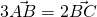# Vectors: Ratio TheoremAs we all know, the Ratio Theorem is given in MF15. But some students struggle to fit in theandevery time. They will then attempt to draw a bit and see it helps. Here’s a solution for students who can never figure out the idea of ratio.

Given, we have that. Notice that you canNOT take a ratio of vectors, so we don’t write it asor! If we simply cross multiply the above, we have. From here,And depending on what vector we are interested, its just a simple manipulation and substitution from here. You will also notice that you can “bump” into the ratio theorem here.

Not readable? Change text.